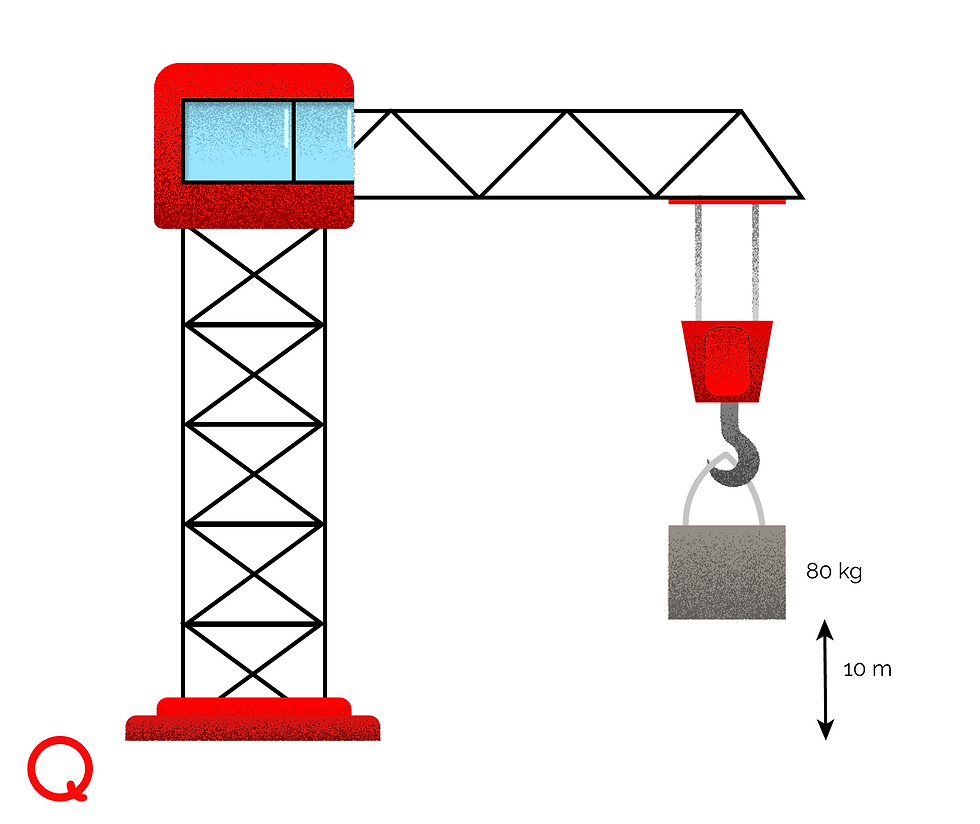cum
top of page
Search

# CSEC Physics: Potential and Kinetic Energy

As we discussed in our previous post on energy, potential energy is stored energy, or energy that an object has by virtue of its position or state of being, while kinetic energy is the energy of movement, or the energy an object has due to being in motion.

Potential Energy

An elastic band (stretched) has potential energy because of its state. Once the force keeping it stretched is removed, its potential energy will be converted to kinetic energy. A ball on top of a hill has potential energy due to its position. Even though it is stationary, it can eventually roll down the hill, converting its potential energy to kinetic energy.

So, it is often useful to think of potential energy as energy that can be converted into another form.

The ball on top of the hill has a specific type of potential energy known as gravitational potential energy. This is due to its position in Earth's gravitational field. To calculate the gravitational potential energy of a given object:

∆Eᴘ = mgh

Where:

∆Eᴘ = gravitational potential energy

m = mass of object (kg)

g = acceleration due to gravity (m/s²)

h = height of object above ground (m)

Note that the m × g part of this equation is the same as weight (w).

Example: A crane lifts an 80 kg mass up by 10 m. What potential energy does it gain? (Take g = 10 m/s²)

∆Eᴘ = mgh

= 80 kg × 10 m/s² × 10 m

= 8000 kgm²/s²

= 8000 JGiven any combination of 3 values from the equation, we can find the missing value.

Example: A ball of mass 300 g on top of a shelf has a potential energy of 50 J. How tall is the shelf?

∆Eᴘ = mgh

Substituting our known values:

50 J = 0.3 kg × 10 m/s² × h

Transposing for h:

h = 50 J/(0.3 kg × 10 m/s²)

= 50 J/3 kgm/s²

h = 16.66 m

Kinetic Energy

A car moving has kinetic energy because it is in motion. In the same way, a rock rolling down the side of a cavern has kinetic energy because it has a velocity that isn't zero.

The kinetic energy of an object is found like this:

Eᴋ = ½mv²

Where:

Eᴋ = kinetic energy (J)

m = mass of object (kg)

v = velocity (m/s)

Example: A tennis ball of 60 g is travelling at 50 m/s. What is its kinetic energy?Eᴋ = ½mv²

= ½ (0.06 kg) × (50 m/s)²

Eᴋ = 75 J